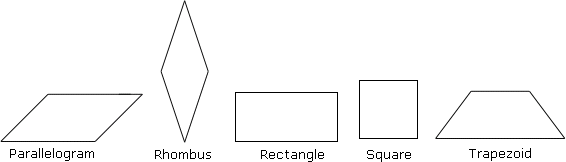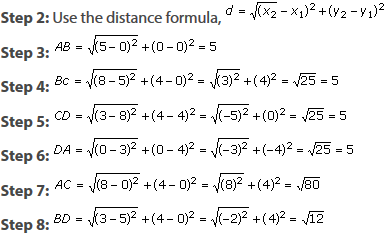A quadrilateral is a four-sided polygon.

Parallelogram: A quadrilateral with two pairs of opposite sides parallel
Rhombus: A parallelogram with four congruent sides.
Rectangle: A parallelogram with four right angles.
Square: A parallelogram with four congruent sides and four right angles.
Trapezoid: A quadrilateral with only one pair of parallel sides.#### Ques: A quadrilateral with vertices (0, 0), (5, 0), (8, 4) and (3, 4) forms a ________.

##### Choices:

A. Rhombus
B. Square
C. Rectangle
D. Trapezoid